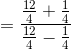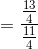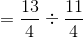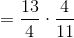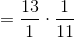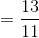# PSAT Math : How to find a complex fraction

## Example Questions

### Example Question #1633 : Psat Mathematics

Simplify: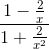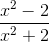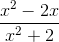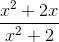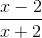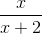Explanation:

Simplify the numerator and the denominator, then divide, as follows: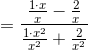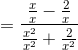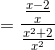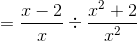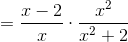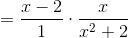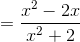### Example Question #1 : Complex Fractions

Evaluate: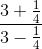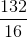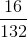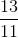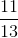Explanation:

Simplify the numerator and the denominator, then divide, as follows: